# Graph Embedding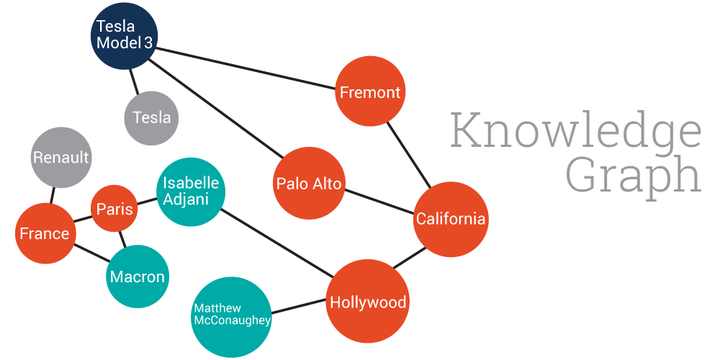Graphs are commonly used in different real-world applications, e.g., social networks are large graphs of people that follow each other. Graph embeddings are the transformation of property graphs to a vector or a set of vectors. Embedding should capture the graph topology, vertex-to-vertex relationship, and other relevant information about graphs, subgraphs, and vertices. The more properties embedder encodes the better results can be retrieved in later tasks.

# Word2vec

Distributed Representations of Words and Phrases and their Compositionality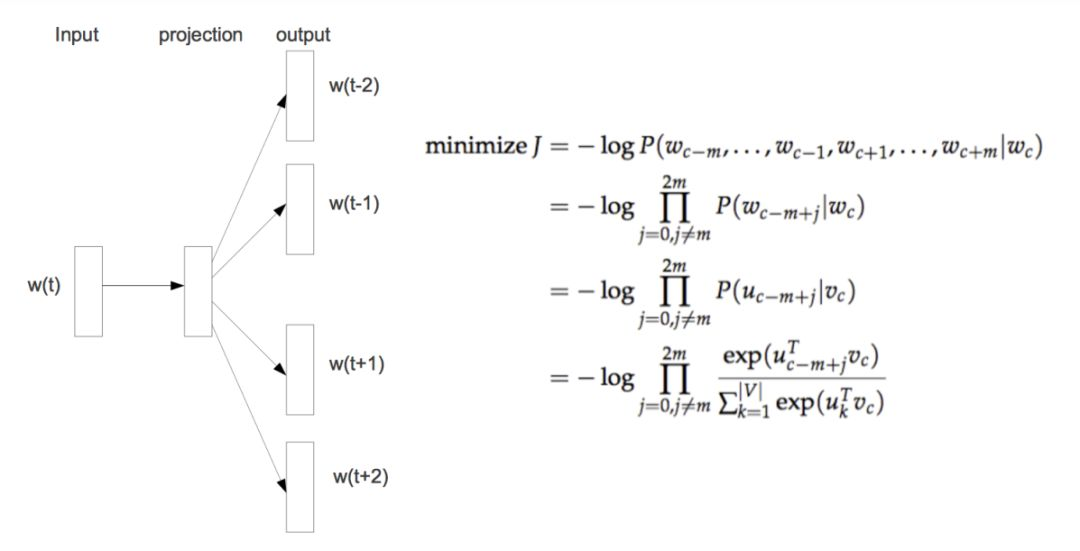Word2vec is one of the most commonly used methods for conversion of text data to the feature vector. Word2vec utilizes the co-occurences of words (n-gram) in document corpus for the computation of the embedding. Additionaly, it formluates the embedding as one of the following problems:

• cbow: predict the word in the middle of a sentence;
• skip-gram: predict the words before and after the given words.

# DeepWalk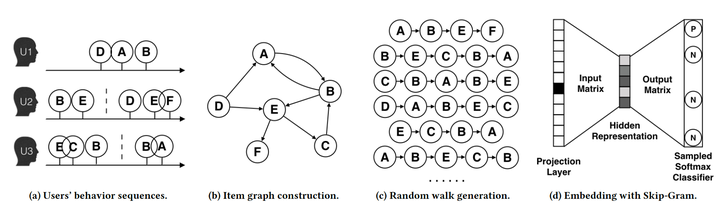DeepWalk: Online Learning of Social Representations proposed in 2014 was one of most important papers regarding ideas for graph embedding. This is how it works:

• starting from a node, start a random walk on the graph;
• feed the sequence of nodes visited on the random walk into a sequence embedding method, e.g., word2vec;

The first step is more important, more specifically, how to define the random walk. In DeepWalk, the transition probability from Node a to Node b is proportional to the weight of edges a to b.

# Line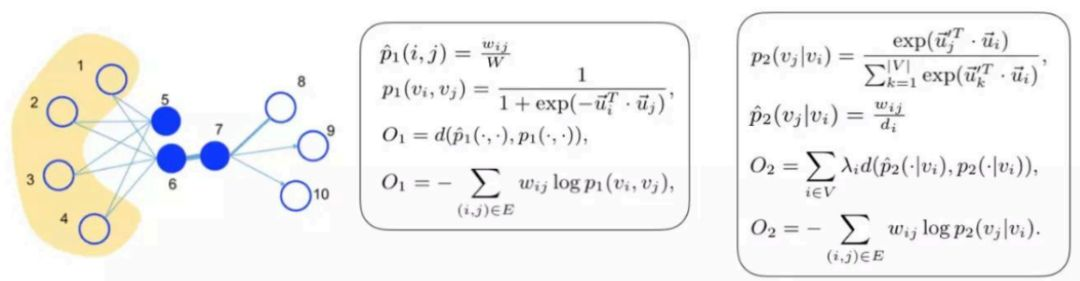Large scale information network embedding

Line considers the similarity of vertices in two aspects:

• 1st order: weights of edges between two vertices;
• 2nd order: how many neighbors do the vertices share.

# GraRep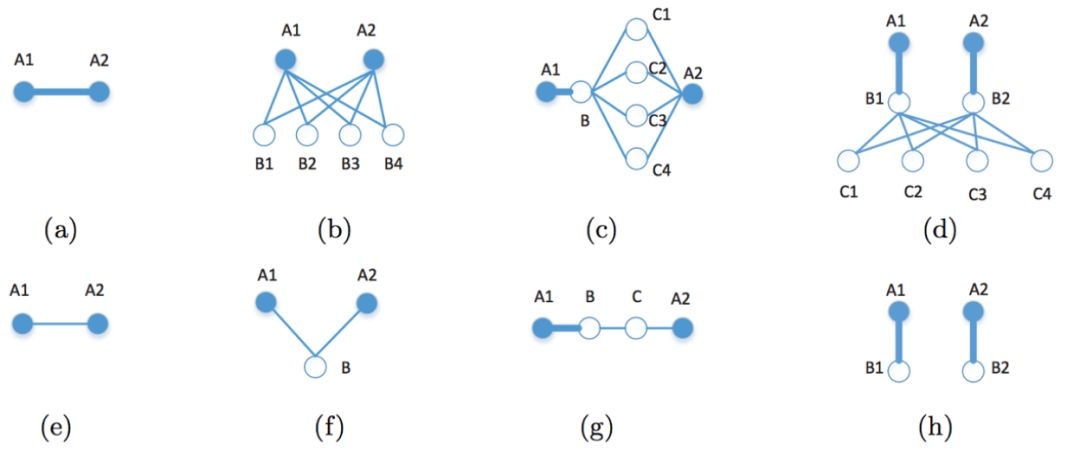Learning Graph Representations with Global Structural

Compared with Line, GraRep considers higher order similarities, where k-order similarity is computed as the probability of traversing from vertex to the other vertex within k-hoops.

# Node2vec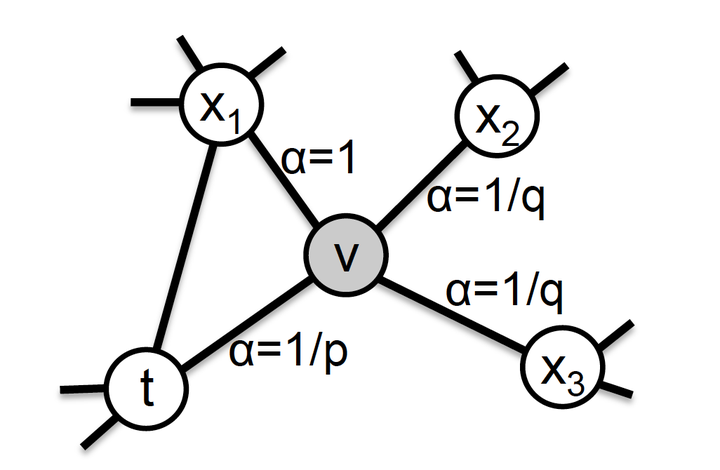Compared with DeepWalk, node2vec: Scalable Feature Learning for Networks improves homophily and structural equivalence by using a different transition probability:

• homophily: nodes close to each other in the graph should have a similar embedding vector;
• structural equivalence: nodes which have a similar neighbor structure should have a similar embedding vector;

BFS prefers homophily and DFS prefers structural equivalence, which could be controlled by transition probablity. More speifically, the transition probablity is computed as: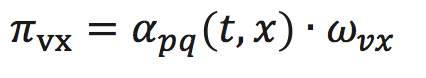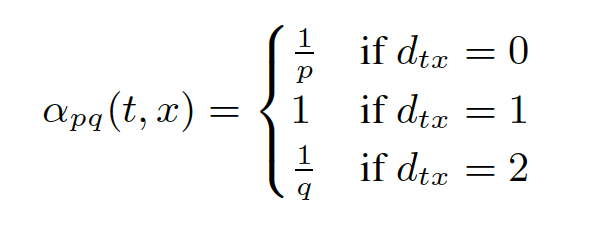where $w_vc$ is the weight of the edge between v and c; $d_tx$ is the distance between t and x. Smaller p encourages BFS; Smaller q encourages DFS.

# Struc2vec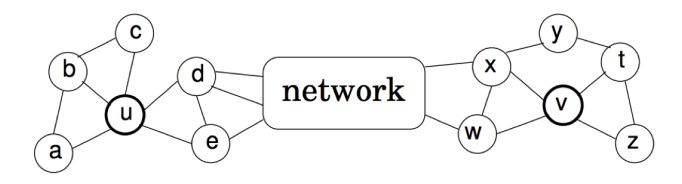struc2vec: Learning Node Representations from Structural Identity

struc2vec proposes that embedding should not consider the direct connectivty of two vertices, but the similarity of structure of two vertices in the network. Note, in many applications, similar vertices do tend to be connected to each other; but this may not hold in all applications.

Let $f_k(u,v)$ is the similarity of two nodes up to k-distance:$g$ is function for measuring similarity of two sequence, $R$ is a traverse sequence.

# GraphSAGE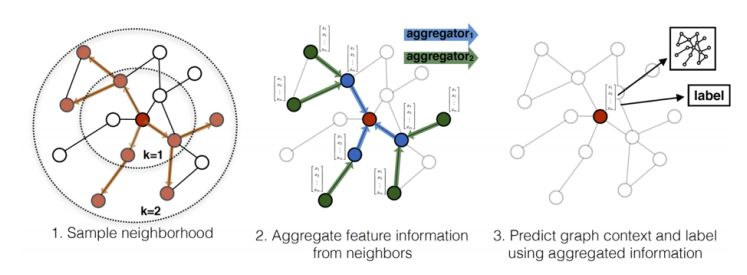Inductive representation learning on large graphs

All the above methods need to be recomputed, when the graph changes. GraphSAGE tries to address this problem by learning aggregate functions instead of the embedding itself. It contains the following steps:

• for each vertex, sample fixed number of neighbors;
• aggregate the neighbors’ features and concatenate them to the current feature;
• repeat for k steps.

The aggregate functions should be orderless. Some typically choices are:

• average pooling
• LSTM
• max pooling
• GCN aggregator

# CANE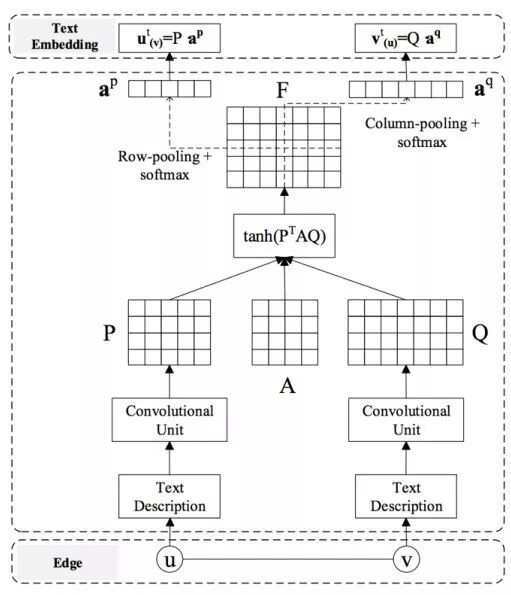Context-Aware Network Embedding for Relation Modeling

CANE considers not only structural similarity but also the property simlarity of vertices and edges. Further, it utilizes word2vec to convert the text associated with each node to a list of feature vectors, then organize those feature vectors as a matrix. CNN is applied to extract matrix features; then row pooling and column pooling is applied to extract attention vector, which is the final embedding.

# SDNE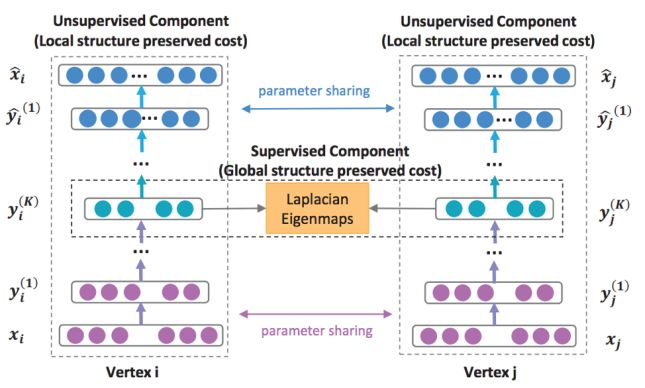Structural Deep Network Embedding

SDNE is based on an auto-encoder. It takes the adjacency matrix as input and requires two conditions:

• that two connected vertices have similar embeddings;
• that the embedding is able to reconstruct the input.

# GraphGAN

GraphGAN: Graph Representation Learning with Generative Adversarial Nets

The basic idea of GraphGAN is 1) the generator generates a new edge in the graph and 2) the discriminator will predict whether an edge exists in the graph or not. In the ideal case, generator will be able to generate edge (based on similarity of embeddings of two vertices) where the discriminator cannot tell whether that edge exists in the original graph or not. The result is, the embedding captures the 1st order information of the graph.

# GraphWave

Learning Structural Node Embeddings via Diffusion Wavelets

# EGES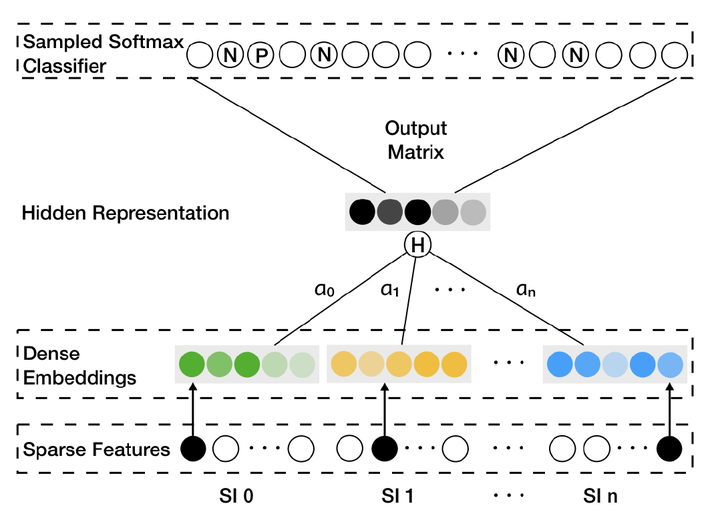Enhanced Graph Embedding with Side Information improves DeepWalk by incorporating the side information. Within the recommendation system cold start is often a challenging but common problem. The situation occurs when a new vertex has few connections to the existing vertices in the graph. This results in DeepWalk cannot generate meaningful embedding for the new vertex. EGES proposes to use side information to address such problems.

The idea of EGES is intuitive: using different side information to generate different embeddings then applying average pool to combine those embeddings.

Written on June 29, 2019